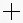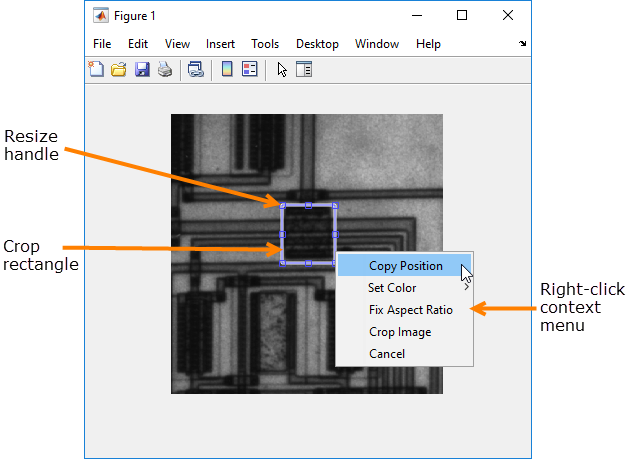# imcrop

## Syntax

``J = imcrop``
``J = imcrop(I)``
``Xout = imcrop(X,cmap)``
``J = imcrop(h)``
``J = imcrop(I,rect)``
``C2 = imcrop(C,rect)``
``Xout = imcrop(X,cmap,rect)``
``J = imcrop(x,y,___)``
``[J,rect2] = imcrop(___)``
``````[x2,y2,___] = imcrop(___)``````

## Description

````J = imcrop` creates an interactive Crop Image tool associated with the image displayed in the current figure. With this syntax and the other interactive syntaxes, the Crop Image tool blocks the MATLAB® command line until you complete the operation. `imcrop` returns the cropped image, `J`.For more information about using the Crop Image tool, see Interactive Behavior.```

example

````J = imcrop(I)` displays the image `I` in a figure window and creates an interactive Crop Image tool associated with the image. `I` can be a grayscale image, a truecolor image, or a logical array.```
````Xout = imcrop(X,cmap)` displays the indexed image `X` in a figure using the colormap `cmap`, and creates an interactive Crop Image tool associated with that image. `imcrop` returns the cropped indexed image, `J`, which also has the colormap `cmap`.```
````J = imcrop(h)` creates an interactive Crop Image tool associated with the image specified by the handle `h`.```

example

````J = imcrop(I,rect)` crops the image `I` according to the position and dimensions specified in the crop rectangle `rect` or an `images.spatialref.Rectangle` object. The crop rectangle, `rect`, is a vector of the form ```[x, y, width, height]``` that specifies the size and position of the cropped image in spatial coordinates. The cropped image includes all pixels in the input image that are completely or partially enclosed by the rectangle.The actual size of the output image does not always correspond exactly with the width and height specified by `rect`. For example, suppose `rect` is `[20 20 40 30]`, using the default spatial coordinate system. The upper left corner of the specified rectangle is the center of the pixel with spatial (x,y) coordinates (20,20). The lower right corner of the rectangle is the center of the pixel with spatial (x,y) coordinates (60,50). The resulting output image has size 31-by-41 pixels, not 30-by-40 pixels.```
````C2 = imcrop(C,rect)` crops the categorical image `C` according to the position and dimensions specified in the crop rectangle `rect`, returning the cropped categorical image `C2`.```

example

````Xout = imcrop(X,cmap,rect)` crops the indexed image `X` with colormap `cmap` according to the position and dimensions specified in the crop rectangle `rect`. Returns the cropped indexed image `X2`.```
````J = imcrop(x,y,___)` crops the input image using a non-default coordinate system, where `x` and `y` specify the image limits in the world coordinate system.```

example

````[J,rect2] = imcrop(___)` returns the position of the cropping rectangle in `rect2`.```
``````[x2,y2,___] = imcrop(___)``` also returns the image limits in `x2` and `y2`.```

## Examples

collapse all

`I = imread('cameraman.tif');`

Open Crop Image tool associated with this image. Specify a variable in which to store the cropped image. The example includes the optional return value `rect` in which `imcrop` returns the four-element position vector of the rectangle you draw.

`[J, rect] = imcrop(I);`

When you move the cursor over the image, it changes to a cross-hairs. The Crop Image tool blocks the MATLAB command line until you complete the operation.

Using the mouse, draw a rectangle over the portion of the image that you want to crop.Perform the crop operation by double-clicking in the crop rectangle or selecting Crop Image on the context menu.The Crop Image tool returns the cropped area in the return variable, `J`. The variable `rect` is the four-element position vector describing the crop rectangle you specified.

`whos`
```Name Size Bytes Class Attributes I 256x256 65536 uint8 J 121x126 15246 uint8 rect 1x4 32 double ```

`I = imread('circuit.tif');`

Crop image, specifying crop rectangle.

`I2 = imcrop(I,[75 68 130 112]);`

Display original image and cropped image.

```subplot(1,2,1) imshow(I) title('Original Image') subplot(1,2,2) imshow(I2) title('Cropped Image')``````I = imread('parkavenue.jpg'); imshow(I)```Specify a target window size as a two-element vector of the form [width, height].

`targetSize = [300 600];`

Create a `Rectangle` object that specifies the spatial extent of the crop window.

`r = centerCropWindow2d(size(I),targetSize);`

Crop the image to the spatial extents. Display the cropped region.

```J = imcrop(I,r); imshow(J)```Load indexed image with its associated map into the workspace.

`load trees`

Crop indexed image, specifying crop rectangle.

`X2 = imcrop(X,map,[30 30 50 75]);`

Display original image and cropped image.

```subplot(1,2,1) imshow(X,map) title('Original Image') subplot(1,2,2) imshow(X2,map) title('Cropped Image')```## Input Arguments

collapse all

Image to be cropped, specified as a numeric, logical, or categorical array. If you specify `rect` as an input argument, `I` can also be logical. Otherwise, `imcrop` calls `imshow` and accepts whatever image classes `imshow` accepts.

Data Types: `single` | `double` | `int8` | `int16` | `uint8` | `uint16` | `logical`

Indexed image to be cropped, specified as an array of integers. If you specify `rect` as an input argument, then `I` can also be logical. Otherwise, `imcrop` calls `imshow` and accepts any image class that `imshow` accepts.

Data Types: `single` | `double` | `int8` | `int16` | `uint8` | `uint16` | `logical`

Colormap associated with indexed image, specified as an c-by-3 numeric matrix.

Data Types: `single` | `double` | `int8` | `int16` | `int32` | `int64` | `uint8` | `uint16` | `uint32` | `uint64` | `logical`

Categorical image, specified as an m-by-n matrix of categorical data.

Data Types: `categorical`

Size and position of the crop rectangle in spatial coordinates, specified as a 4-element numeric vector of the form `[xmin ymin width height]` or a `images.spatialref.Rectangle` object.

Data Types: `single` | `double` | `int8` | `int16` | `int32` | `int64` | `uint8` | `uint16` | `uint32` | `uint64`

Input image, specified as a handle to a figure, axes, uipanel, or image graphics object. If `h` is an axes or figure handle, imcrop uses the first image returned by `findobj(H,'Type','image')`.

Image limits in world coordinates along the x- or y-dimension, specified as a 2-element numeric vector of the form `[min max]`. The values of `x` and `y` set the image `XData` and `YData`. The data type of `x` and `y` must match.

The second element of `x` and `y` must be greater than the first element. `imcrop` does not support coordinate systems with inverted axes.

Data Types: `single` | `double` | `int8` | `int16` | `int32` | `int64` | `uint8` | `uint16` | `uint32` | `uint64`

## Output Arguments

collapse all

Cropped image, returned as a numeric array.

• If you specify an input image, then the output image has the same class as the input image.

• If you do not specify an input image, then the output image generally has the same class as the input image. However, if the input image is `int16` or `single`, then the output image is `double`.

Cropped categorical image, returned as a categorical matrix.

Cropped indexed image, returned as a numeric array.

Size and position of the crop rectangle, returned as a 4-element numeric vector of the form `[xmin ymin width height]`.

Image limits in the x or y direction, returned as a 2-element numeric vector of the form `[min max]`. If you specify image limits `x` and `y`, then `x2` and `y2` are equal to these values. Otherwise, `x2` and `y2` are equal to the original image `XData` and `YData`.

collapse all

### Interactive Behavior

The Crop Image tool is a moveable, resizeable rectangle that you can position over the image and perform the crop operation interactively using the mouse.

When the Crop Image tool is active in a figure, the pointer changes to cross hairswhen you move it over the target image. Using the mouse, you specify the crop rectangle by clicking and dragging the mouse. You can move or resize the crop rectangle using the mouse. When you are finished sizing and positioning the crop rectangle, create the cropped image by double-clicking the left mouse button. You can also choose Crop Image from the context menu. The following figure illustrates the Crop Image tool with the context menu displayed.Interactive BehaviorDescription
Delete the Crop Image tool.

Press Backspace, Escape or Delete, or right-click inside the crop rectangle and select Cancel from the context menu.

Note: If you delete the ROI, the function returns empty values.

Resize the Crop Image tool.Select any of the resize handles on the crop rectangle. The pointer changes to a double-headed arrow. Click and drag the mouse to resize the crop rectangle.
Move the Crop Image tool.Move the pointer inside the boundary of the crop rectangle. The pointer changes to a fleur shape. Click and drag the mouse to move the rectangle over the image.
Change the color used to display the crop rectangle.Right-click inside the boundary of the crop rectangle and select Set Color from the context menu.
Crop the image.

Double-click the left mouse button or right-click inside the boundary of the crop rectangle and select Crop Image from the context menu.

Retrieve the coordinates of the crop rectangle.Right-click inside the boundary of the crop rectangle and select Copy Position from the context menu. `imcrop` copies a 4-element position vector (`[xmin ymin width height]`) to the clipboard.